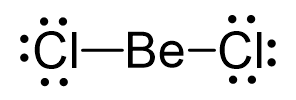## Examples

Be is the central atom, so we can draw the skeletal structure:There is a total of 2 + 2×7 = 16 valence electrons, and we use four of them to make the bonds. The remaining 12 go on the Cl atoms as lone pairs:The steric number is 2, and there are no lone pairs on the central atom. Therefore, both the electron and molecular geometries are linear.

Steric number 2 corresponds to sp-hybridization where the bond angles are 180o.